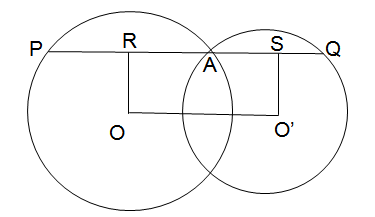### (Sample Printed worksheet)

1)AOC is a diameter of the circle and arc AXB = (1/3) arc BYC. Find ∠BOC.2) AB is diameter of the circle. If A and B are connected to E, circle is intersected at C and D respectively. If AB = 16 cm and CD = 8 cm, find ∠AEB.3) Two circles with radii of 4 and 10 are drawn with the same centre. The smaller inner circle is painted yellow, and the part outside the smaller circle and inside the larger circle is painted pink. What is the ratio of the areas painted pink to the area painted yellow?
4)Given a circle, a circumscribed square is one that completely encloses the circle, and an inscribed square is completely enclosed by the circle, as shown belowIf the the difference in the areas of the two squares is 28 sq. m., then what is the area of the circle? Assume π =
 22 7
.
 5) if CD = 1 cm, OA = 25 cm and OD is perpendicular to AB, then AB = ?### Fill in the blanks

6)AC is diameter of the triangle and BD is perpendicular to AC. If AB = 25 cm and BC = 9 cm, find the length of BD.7) ABCD is a square. Two arcs are drawn with A and B as centers, and radius equal to the side of square. If arcs intersects at point E, the angle ∠ACE = �.### Choose correct answer(s) from given choice

8)If ∠DAB = 71� and ∠ABD = 59�, find the value of ∠ACB.a. 50� b. 45� c. 60� d. 55�
9)Two circles with centres O and O′ intersect at two points A and B. A line PQ is
drawn parallel to OO′ through A(or B) intersecting the circles at P and Q. Find the ratio OO′:PQ.
 a. 1:1 b. 2:3 c. 2:1 d. 1:2
10)ABCD is a cyclic quadrilateral such that AB is a diameter of the circle circumscribing it and ∠ADC = 110�, then find the value of ∠BAC.
 a. 15� b. 20� c. 25� d. 10�

1) 135�
 Step 1 The ratio of the arc length to the circumference will be the same as the angle subtended by the arc/chord to the angle in a full circle (360�) Step 2 Here we know that arc AXB = (1/3) arc BYC This means ∠BOA = (1/3) ∠BOC Step 3 Also AOC is the diameter, so ∠BOA + ∠BOC = 180� (1/3) ∠BOC + ∠BOC = 180� ∠BOC = �

2) 60�

3) 21:4

4) 44 sq. m.

5) 14 cm
Step 1
We know the following
OA and OD are the radii of the circle. Therefore OA=OD
OD is perpendicular to AB. Segment OC is also therefore perpendicular to AB. ∠OCA = 90�
Also, the point C divides AB into two. So AC=CB =
 AB 2

We also know that CD = OD - OC = OA - OC
Step 2
OAC is a right-angle triangle - therefore AC2 + OC2 = OA2
From this, the relation between AB, CD and OA can be given as
- (
 AB 2
)2 + (OA - CD)2 = OA2
Step 3
Here we know CD = 1 and OA = 25
Substituting and simplifying in the equation we get the value of AB = 14 cm

6)
7)
8) a. 50�
 Step 1 The angle subtended by a chord to two points on the circumference are equal, if the two points are on the same side of the chord Step 2 This means that ∠ACB = ∠BDA Step 3 Now, ABC and ABD are triangles, so the sum of the internal angles is 180� Step 4 For triangle ABD, this means ∠DAB + ∠ABD + ∠BDA = 180� 71 + 59 + ∠BDA = 180 ∠BDA = 180 � (71 + 59) ∠BDA = 50�

9) d. 1:2
 Step 1 Consider the image belowWe see that the line drawn at point O perpendicular to OO′ meets the line PQ at a point R A similar line drawn at O′ meets PQ at a point S Now PA is a chord, and OR is a line perpendicular to it drawn from the circle OR therefore bisects OA, and PR = RA PA = 2 RA Step 2 By the same reasoning AQ = 2 AS PQ = PA + AQ PQ = 2RA + 2 AS PQ = 2 (RA + AS) But RA + AS is the same as OO′ Therefore PQ = 2 OO′ The ratio of PQ:OO′ is therefore 2:1

10) b. 20�
 Step 1 Consider a cyclic quadrilateralWe know the following - The sum of the opposite angles is 180� i.e ∠ADC + ∠ABC = 180� - The angle subtended by a diameter to a point on the circumference = 90� Step 2 We know that ∠ADC = 110� ∠ADC + ∠ABC = 110� + ∠ABC = 180� ∠ABC = 70� Step 3 Now consider the triangle formed by points A,B,C. We need to find ∠BAC We know ∠ABC + ∠BAC + ∠ACB = 180� (as it is a triangle) ∠ABC = 70, and ∠ACB is the angle subtended by the diameter to a point at the circumference, C Therefore ∠ACB = 90� From these, we get 70� + ∠BAC + 90� = 180� ∠BAC = 180� - (70� + 90�) = 20�